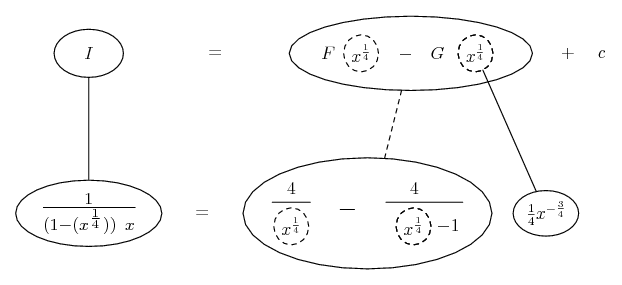# Thread: Problem with the answer provided by wolfram alpha

1. ## Problem with the answer provided by wolfram alpha

I am having a problem with evaluating the integral so i put the question in it and i got a answer but i am not sure of his steps

$\displaystyle \int \frac{1}{x(1-x^{\frac{1}{4}})}$

http://www.wolframalpha.com/input/?i=integrate+dx%2F+(x(1-x^(1%2F4)))

I didnt understand how he substituted 'u' in the equation.

2. Have you considered the sustitution $\displaystyle u=x^{1/4}$?

-Kevin C.

3. $\displaystyle \int{\frac{1}{x(1 - x^{\frac{1}{4}})}\,dx}$

Let $\displaystyle u = x^{\frac{1}{4}}$.

Then $\displaystyle \frac{du}{dx} = \frac{1}{4}x^{-\frac{3}{4}} = \frac{1}{4x^{\frac{3}{4}}}$.

So $\displaystyle dx = 4x^{\frac{3}{4}}\,du$.

Can you see that $\displaystyle \frac{dx}{x} = \frac{4x^{\frac{3}{4}}\,du}{x}$

$\displaystyle = 4x^{-\frac{1}{4}}\,du$

$\displaystyle = \frac{4\,du}{x^{\frac{1}{4}}}$

$\displaystyle = \frac{4\,du}{u}$.

So the integral becomes

$\displaystyle 4\int{\frac{1}{u(1 - u)}\,du}$.

Can you go from here?

4. Same thing dressed (only very) slightly different...

$\displaystyle \frac{1}{(1 - (x^{\frac{1}{4}}))\ x}$

$\displaystyle =\frac{1}{(1 - (x^{\frac{1}{4}}))\ (x^{\frac{1}{4}})^4}$

$\displaystyle =\frac{1}{(1 - (x^{\frac{1}{4}}))\ (x^{\frac{1}{4}})^4}\ \ \frac{\frac{1}{4}(x^{\frac{1}{4}})^{-3}}{\frac{1}{4}(x^{\frac{1}{4}})^{-3}}$

$\displaystyle =\frac{1}{(1 - (x^{\frac{1}{4}}))\ (x^{\frac{1}{4}})^4\ \frac{1}{4}(x^{\frac{1}{4}})^{-3}}\ \ \frac{1}{4}(x^{\frac{1}{4}})^{-3}$

$\displaystyle =\frac{4}{(1 - (x^{\frac{1}{4}}))\ (x^{\frac{1}{4}})}\ \ \frac{1}{4}(x^{\frac{1}{4}})^{-3}$

$\displaystyle =\frac{4}{(1 - (x^{\frac{1}{4}}))\ (x^{\frac{1}{4}})}\ \ d(x^{\frac{1}{4}})$

Edit:

Then partial fractions, or else this trick...

$\displaystyle =4\ [\ \frac{1 - (x^{\frac{1}{4}}) + (x^{\frac{1}{4}})}{(1 - (x^{\frac{1}{4}}))\ (x^{\frac{1}{4}})}\ ]\ \ d(x^{\frac{1}{4}})$

$\displaystyle =4\ [\ \frac{(1 - (x^{\frac{1}{4}}))}{(1 - (x^{\frac{1}{4}}))\ x^{\frac{1}{4}}} + \frac{x^{\frac{1}{4}}}{(1 - (x^{\frac{1}{4}}))\ x^{\frac{1}{4}}}\ ]\ \ d(x^{\frac{1}{4}})$

$\displaystyle =4\ [\ \frac{1}{(x^{\frac{1}{4}})} + \frac{1}{1 - (x^{\frac{1}{4}})}\ ]\ \ d(x^{\frac{1}{4}})$

Also, just in case an overview helps...... where... is the chain rule. Straight continuous lines differentiate downwards (integrate up) with respect to x, and the straight dashed line similarly but with respect to the dashed balloon expression (the inner function of the composite which is subject to the chain rule).

__________________________________________

Don't integrate - balloontegrate!

Balloon Calculus; standard integrals, derivatives and methods

Balloon Calculus Drawing with LaTeX and Asymptote!

#### Search Tags

alpha, answer, problem, provided, wolfram